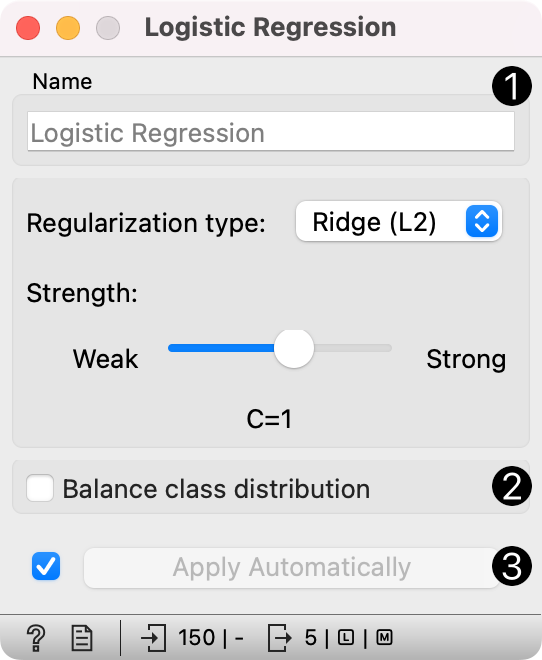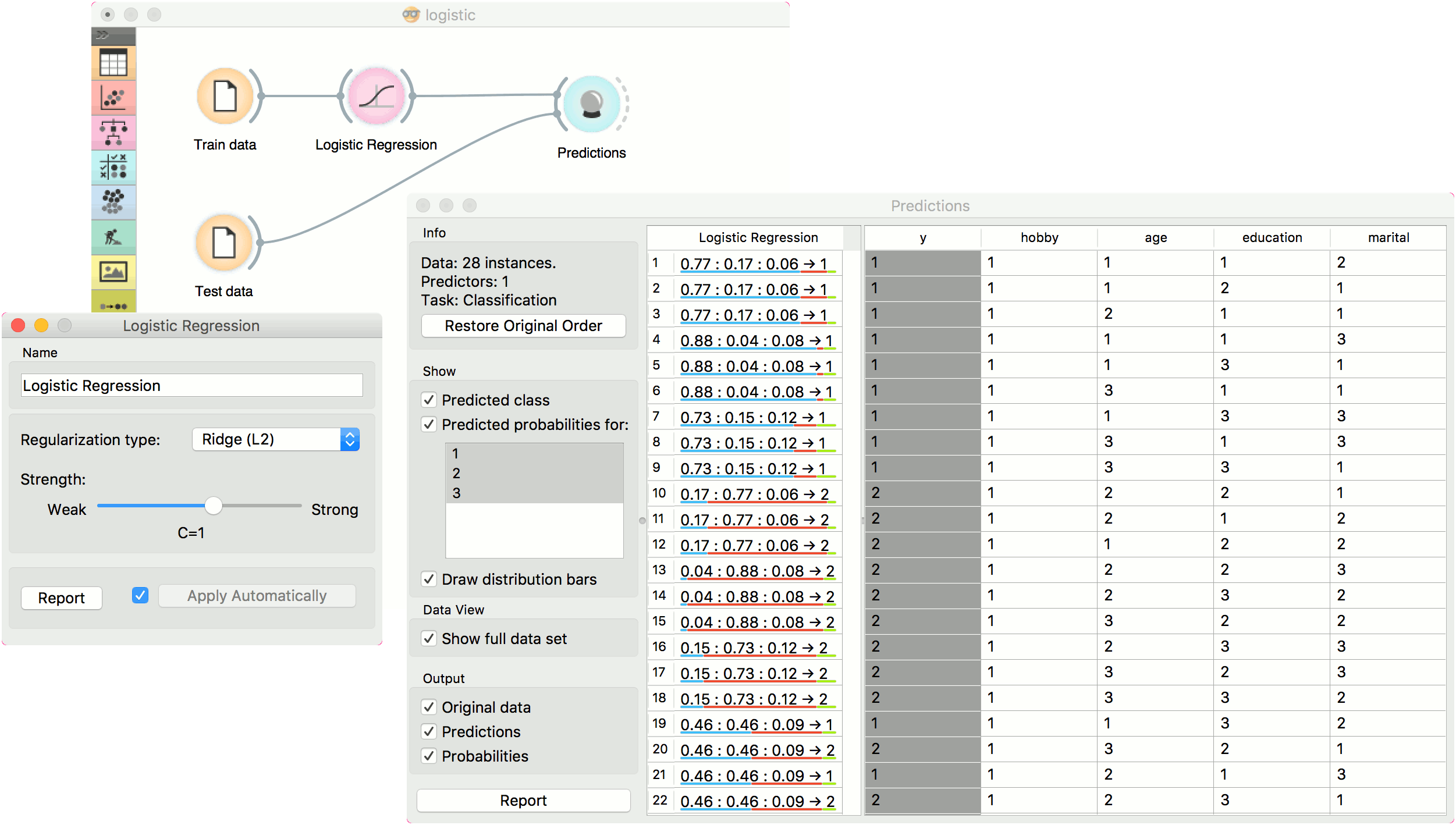# Logistic Regression

The logistic regression classification algorithm with LASSO (L1) or ridge (L2) regularization.

Inputs

• Data: input dataset
• Preprocessor: preprocessing method(s)

Outputs

• Learner: logistic regression learning algorithm
• Model: trained model
• Coefficients: logistic regression coefficients

Logistic Regression learns a Logistic Regression model from the data. It only works for classification tasks.1. A name under which the learner appears in other widgets. The default name is “Logistic Regression”.
2. Regularization type (either L1 or L2). Set the cost strength (default is C=1).
3. Press Apply to commit changes. If Apply Automatically is ticked, changes will be communicated automatically.

## Preprocessing

Logistic Regression uses default preprocessing when no other preprocessors are given. It executes them in the following order:

• removes instances with unknown target values
• continuizes categorical variables (with one-hot-encoding)
• removes empty columns
• imputes missing values with mean values

To remove default preprocessing, connect an empty Preprocess widget to the learner.

## Feature Scoring

Logistic Regression can be used with Rank for feature scoring. See Learners as Scorers for an example.

## Example

The widget is used just as any other widget for inducing a classifier. This is an example demonstrating prediction results with logistic regression on the hayes-roth dataset. We first load hayes-roth_learn in the File widget and pass the data to Logistic Regression. Then we pass the trained model to Predictions.

Now we want to predict class value on a new dataset. We load hayes-roth_test in the second File widget and connect it to Predictions. We can now observe class values predicted with Logistic Regression directly in Predictions.